# Ms. Chang 4th Grade- Divide with partial quotients

##By Ms Chang

Find whole-number quotients and remainders with up to four-digit dividends and one-digit divisors, using strategies based on place value, the properties of operations, and/or the relationship between multiplication and division# Four-Digit by One Digit Division (Equal Groups/Partial Quotients): Grade 4

##By University Of Houstan Mathematics Education

Find whole-number quotients and remainders with up to four-digit dividends and one-digit divisors, using strategies based on place value# [4.NBT.6-1.0] Four digit division - Common Core Standard

##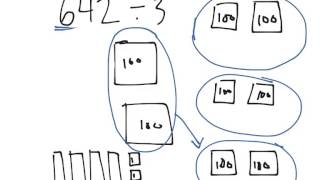By Freckle education

Find whole-number quotients and remainders with up to four-digit dividends and one-digit divisors, using strategies based on place value, the properties of operations, and/or the relationship between multiplication and division# Evaluating Logarithms | MathHelp.com

##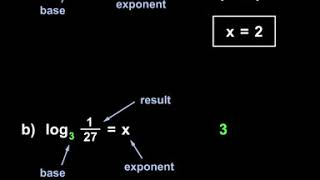By MathHelp.com

In this example, notice that we have a polynomial divided by a binomial, and our binomial is in the form of an x term minus a constant term, or x – c. In this situation, instead of having to use long division, like we did in the previous lesson, we can divide the polynomials using synthetic division, which is a much more efficient method. Here’s how it works. We start by finding the value of c. Since –c = -3, we know that c = 3. Next, we put the value of c inside a box, so we put the 3 inside a box. It’s very important to understand that the number that goes inside the box always uses the opposite sign as the constant term in the binomial. In other words, since the constant term in the binomial is -3, the number that goes inside the box, is positive 3. Next, we write the coefficients of the dividend, which are 2, -7, 4, and 5. Be very careful with your signs. Now, we’re ready to start our synthetic division. First, we bring down the 2. Next, we multiply the 3 in the box times 2 to get 6, and we put the 6 under the -7. Next, we add -7 + 6 to get -1. Next, we multiply the 3 in the box times -1 to get -3, and we put the -3 under the 4. Next, we add 4 + -3 to get 1. Next, we multiply the 3 in the box times 1 to get 3, and we put the 3 under the 5. Finally, we add 5 + 3 to get 8. Now, notice that we have a 2, -1, 1, and 8 in the bottom row of our synthetic division. These values will give us our answer: the first 3 numbers represent the coefficients of the quotient, and the last number is the remainder. And it’s important to understand that our answer will be one degree less than the dividend. In other words, since our dividend starts with x cubed, and we’re dividing by x, our answer will start with x squared. So our answer is 2x squared – 1x + 1 + 8 over x – 3. Notice that we always use descending order of powers in our quotient. In this case x squared, x, and the constant. Finally, remember that we add the remainder over the divisor, just like we did in the previous lesson on long division, and we have our answer. It’s important to understand that we’ll get the same answer whether we use synthetic division or long division. However, synthetic division is much faster.# Learn Division for Kids - 2nd and 3rd Grade Math Video

##By Lumos Learning

Check out the NEW Math Game we made at https://www.MageMath.com/​ It is a full video game called Mage Math that helps kids build confidence in math while having tons of fun.# [5.NBT.6-1.0] Multi-digit divisors - Common Core Standard - Word Problem

##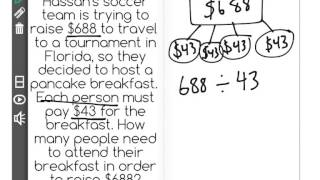By Front Row

Discover more Common Core Math at https://www.frontrowed.com Find whole-number quotients of whole numbers with up to four-digit dividends and two-digit divisors, using strategies based on place value, the properties of operations, and/or the relationship between multiplication and division Front Row is a free, adaptive, Common Core aligned math program for teachers and students in kindergarten through eighth grade. Front Row allows students to practice math at their own pace - learning advanced concepts when they're ready and receiving remediation when they struggle. Front Row provides teachers with access to a detailed data dashboard and weekly email reports that show which standards are causing students difficulty, what small groups can be formed for interventions, and how their students are progressing in math.Discover more Common Core Math at https://www.frontrowed.com# Synthetic Division | MathHelp.com

##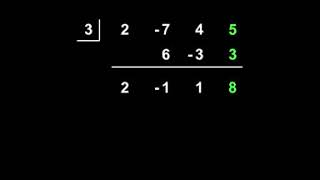By MathHelp.com

In this example, it’s tempting to divide x squared + 5x – 6 by x + 1 by first factoring x squared + 5x – 6. The factors of -6 that add to positive 5 are +6 and -1, so we have x + 6 times x – 1 over x + 1. Notice, however, that nothing cancels. In this situation, we need a different method of dividing the polynomials, so we use long division. In other words, we rewrite x squared + 5x – 6 divided by x + 1 as x + 1 divided into x squared + 5x – 6. Now, our first step in the long division is to determine how many times x goes into x squared. Since x goes into x squared x times, we write an x above the x squared, just like we do with regular long division. Next, we multiply the x times the x + 1 in the divisor to get x squared + x, and we write the x squared + x underneath the x squared + 5x. Next, we subtract x squared + x from x squared + 5x. And watch out for this step: it’s an area where most of the common mistakes in these types of problems are made. Instead of subtracting, I would change the sign of each term in x squared + x, so we have negative x squared + negative x, then add the columns. So we have x squared + negative x squared, which cancels out, and positive 5x + negative x, which is positive 4x. Next, we bring down the -6, in regular long division. Now, we need to determine how many times x goes into 4x. Since x goes into 4x 4 times, we write a positive 4 in our answer. Next, we multiply positive 4 times x + 1 to get 4x + 4, and we write the 4x + 4 underneath the 4x – 6. Next, we subtract 4x + 4 from 4x – 6. In other words, we change the signs on 4x + 4 to -4x + -4, and we add. 4x + -4x cancels out, and -6 + -4 is -10. And since there are no other numbers to bring down, we have a remainder of -10. Finally, remember from the previous example that we add the remainder over the divisor to the quotient. In other words, we add -10 over x + 1 to x + 4, and we have x + 4 + -10 over x + 1. So x squared + 5x – 6 divided by x + 1 simplifies to x + 4 + -10 over x + 1.# Estimating Quotients | MathHelp.com

##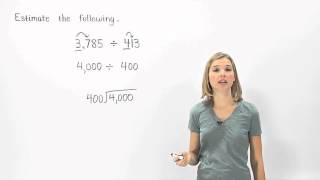By MathHelp.com

This lesson covers commutative property of multiplication, which states that a x b = b x a. For example, 10 x 4 = 4 x 10.# Math Antics - Basic Division

##By Lumos Learning# Polynomial Long Division | MathHelp.com

##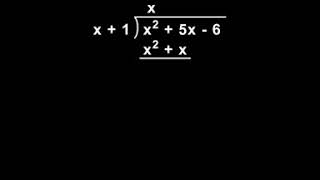By MathHelp.com# Math Antics - What Is Arithmetic?

##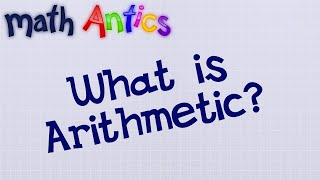By mathantics# 10 - The Remainder Theorem of Synthetic Division & Polynomial Long Division - Part 1

##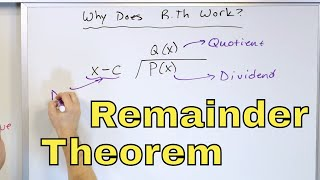By Math and Science

Quality Math And Science Videos that feature step-by-step example problems!# Multiplying and Dividing Integers

##By Anywhere Math# Writing Expressions

##By Anywhere Math# 06 - Proving the Logarithm (Log) Rules - Understand Logarithm Rules & Laws of Logs

##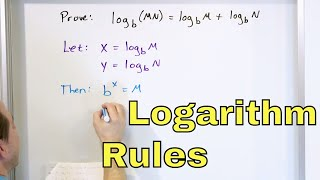By Math and Science

Quality Math And Science Videos that feature step-by-step example problems!# 18 - Properties of Logarithms (Log x) - Part 1 - Laws of Logs - Calculate Logs & Simplify

##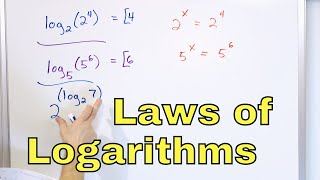By Math and Science

Quality Math And Science Videos that feature step-by-step example problems!